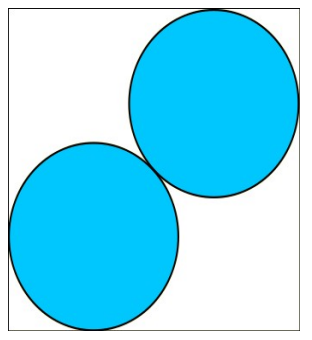시간 제한메모리 제한제출정답맞힌 사람정답 비율
4 초 128 MB267694619.658%

## 문제

We will consider a convex polygon with N vertices. We wish to find the maximum radius R such that two circles of radius R can be placed entirely inside the polygon without overlapping.

## 입력

The first line of input contains the number N. Each of the next N lines contains a pair of integers xi, yi – representing the coordinates of the ith point, separated by space.

## 출력

You should output a single number R – the desired radius. Output R with a precision of 3 decimals. You will pass a test if the output differs from the true answer by at most 0.001.

## 제한

• 3 ≤ N ≤ 50000
• -107 ≤ xi ≤ 107
• -107 ≤ yi ≤ 107
• The points are given in trigonometric (anti-clockwise) order.

## 예제 입력 1

4
0 0
1 0
1 1
0 1


## 예제 출력 1

0.293


## 예제 입력 2

4
0 0
3 0
3 1
0 1


## 예제 출력 2

0.500


## 예제 입력 3

6
0 0
8 0
8 6
4 8
2 8
0 4


## 예제 출력 3

2.189


## 힌트

The maximum radius is obtained when the centers of the two circles are placed on one of the square's diagonals. The radius can be calculated exactly and it is:

$$\dfrac{\sqrt{2}}{2 \times (1 + \sqrt{2})} \approx 0.293$$